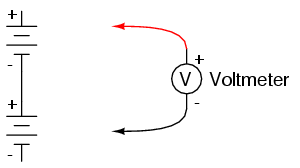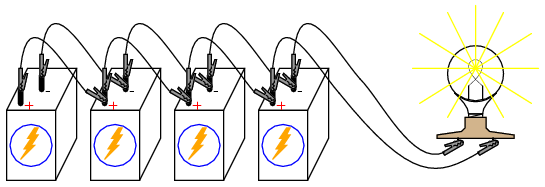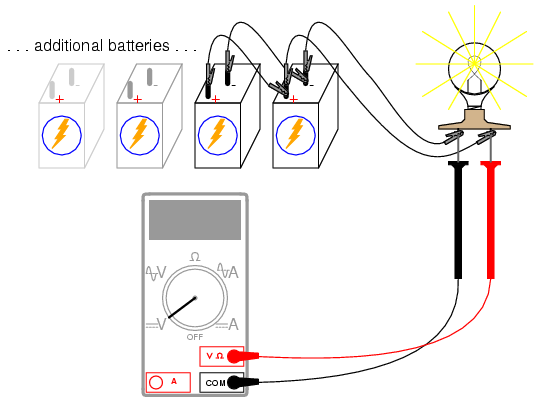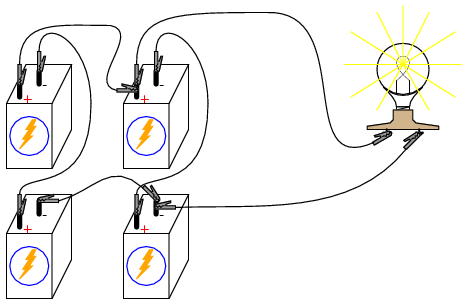# battery experiment

## Series batteries

PARTS AND MATERIALS

Two 6-volt batteries
One 9-volt battery

Actually, any size batteries will suffice for this experiment, but it is recommended to have at least two different voltages available to make it more interesting.

LEARNING OBJECTIVES

How to connect batteries to obtain different voltage levels

SCHEMATIC DIAGRAMILLUSTRATIONINSTRUCTIONS

Connecting components in series means to connect them in-line with each other, so that there is but a single path for electrons to flow through them all. If you connect batteries so that the positive of one connects to the negative of the other, you will find that their respective voltages add. Measure the voltage across each battery individually as they are connected, then measure the total voltage across them both, like this:Try connecting batteries of different sizes in series with each other, for instance a 6-volt battery with a 9-volt battery. What is the total voltage in this case? Try reversing the terminal connections of just one of these batteries, so that they are opposing each other like this:How does the total voltage compare in this situation to the previous one with both batteries “aiding?” Note the polarity of the total voltage as indicated by the voltmeter indication and test probe orientation. Remember, if the meter’s digital indication is a positive number, the red probe is positive (+) and the black probe negative (-); if the indication is a negative number, the polarity is “backward” (red=negative, black=positive). Analog meters simply will not read properly if reverse-connected, because the needle tries to move the wrong direction (left instead of right). Can you predict what the overall voltage polarity will be, knowing the polarities of the individual batteries and their respective strengths?

## Parallel batteries

PARTS AND MATERIALS

Four 6-volt batteries
12-volt light bulb, 25 or 50 watt
Lamp socket

High-wattage 12-volt lamps may be purchased from recreational vehicle (RV) and boating supply stores. Common sizes are 25 watt and 50 watt. This lamp will be used as a “heavy” load for your batteries (heavy load = one that draws substantial current).

A regular household (120 volt) lamp socket will work just fine for these low-voltage “RV” lamps.

LEARNING OBJECTIVES

Voltage source regulation
Boosting current capacity through parallel connections

SCHEMATIC DIAGRAMILLUSTRATIONINSTRUCTIONS

Begin this experiment by connecting one 6-volt battery to the lamp. The lamp, designed to operate on 12 volts, should glow dimly when powered by the 6-volt battery. Use your voltmeter to read voltage across the lamp like this:The voltmeter should register a voltage lower than the usual voltage of the battery. If you use your voltmeter to read the voltage directly at the battery terminals, you will measure a low voltage there as well. Why is this? The large current drawn by the high-power lamp causes the voltage at the battery terminals to “sag” or “droop,” due to voltage dropped across resistance internal to the battery.

We may overcome this problem by connecting batteries in parallel with each other, so that each battery only has to supply a fraction of the total current demanded by the lamp. arallel connections involve making all the positive (+) battery terminals electrically common to each other by connection through jumper wires, and all negative (-) terminals common to each other as well. Add one battery at a time in parallel, noting the lamp voltage with the addition of each new, parallel-connected battery:There should also be a noticeable difference in light intensity as the voltage “sag” is improved.

Try measuring the current of one battery and comparing it to the total current (light bulb current). Shown here is the easiest way to measure single-battery current:By breaking the circuit for just one battery, and inserting our ammeter within that break, we intercept the current of that one battery and are therefore able to measure it. Measuring total current involves a similar procedure: make a break somewhere in the path that total current must take, then insert the ammeter within than break:Note the difference in current between the single-battery and total measurements.

To obtain maximum brightness from the light bulb, a series-parallel connection is required. Two 6-volt batteries connected series-aiding will provide 12 volts. Connecting two of these series-connected battery pairs in parallel improves their current-sourcing ability for minimum voltage sag:Related

COMMENT songs

1. sharif

I would likes to thank you
Sharif